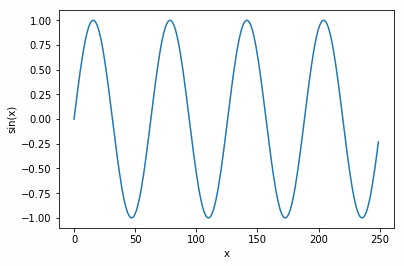﻿ How to Add Subplots in Matplotlib – BMC Blogs

Start by plotting one chart onto the chart surface.

• Use plt.axes(), with no arguments. Matplotlib will then autofit the chart to our data.
• The function np.arange(0,25,0.1) creates 250 numbers ranging from 0 to 25 in increments of 0.1.
• The y axis will range between 1 and -1 since the sin function np.sin(x) ranges between 1 and -1.
• Annotate the chart by labelling each axis with plt.ylabel(‘sin(x)’) and plt.xlabel(‘x’).
```import numpy as np
import matplotlib.pyplot as plt

x = np.arange(0, 25,0.1)

axis1 = plt.axes()
plt.ylabel('sin(x)')
plt.xlabel('x')
axis1.plot(np.sin(x))
```

That results in this chart:### Vertically stacked figures

Now, plot two charts, one stacked on top of the other. Use plt.subplots(2). Note that we plot sin(x) in the top chart and cos(x) in the bottom to avoid graphing the same data twice.

```import numpy as np
import matplotlib.pyplot as plt

x = np.arange(0, 25,0.1)
fig, axis = plt.subplots(2)

plt.ylabel('sin(x)')
plt.xlabel('x')
axis.plot(np.sin(x))
axis.plot(np.cos(x))
```

That results in:### Horizontal Side by Side Figures

• fig, axis = plt.subplots(1,2,figsize=(15,5)) meaning 1 row and 2 columns. Add figsize meaning width and heights, respectfully.
• Note: There is something not clear here. The Matplotlib documentation says this is given in inches, but it’s not, as the chart below will show the same size regardless of the size of your monitor—and why would a system used by people around the world not use the metric system? This seems to be a relative size. So, we may have to call this a documentation bug for now. (Write to us at blogs@bmc.com if you know the answer to this.)
```import numpy as np
import matplotlib.pyplot as plt

x = np.arange(0, 25,0.1)
fig, axis = plt.subplots(1,2,figsize=(15,5))

plt.ylabel('sin(x)')
plt.xlabel('x')
axis.plot(np.sin(x))
axis.plot(np.cos(x))

```

This results in the two charts placed side-by-side but spread farther apart.### Automate workflows to simplify your big data lifecycle

In this e-book, you’ll learn how you can automate your entire big data lifecycle from end to end—and cloud to cloud—to deliver insights more quickly, easily, and reliably.

Last updated: 09/05/2019

These postings are my own and do not necessarily represent BMC's position, strategies, or opinion.

See an error or have a suggestion? Please let us know by emailing blogs@bmc.com.

### BMC Bring the A-Game

From core to cloud to edge, BMC delivers the software and services that enable nearly 10,000 global customers, including 84% of the Forbes Global 100, to thrive in their ongoing evolution to an Autonomous Digital Enterprise.